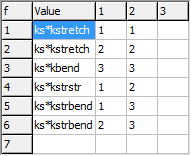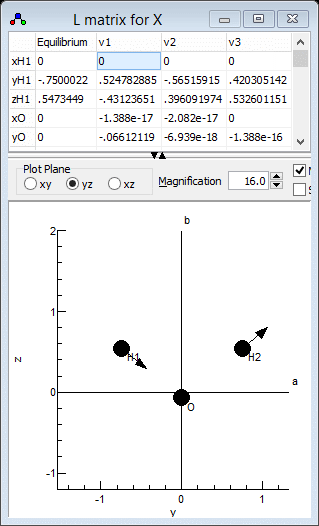Molecule Types Vibrational Structure Force Field Analysis

# Worked Example - Refining an ab initio force field for H2O

This example presents an alternative approach to setting up a force field calculation - using an ab initio calculation to generate the geometry and force field, and then adjusting these to improve the agreement with observed values. The ab initio calculations used here is from the worked example Predicting the Photoelectron Spectrum of H2O using ab initio Calculations. See also the worked example Setting up a simple force field analysis for H2O, which sets up a similar file, though without using ab initio calculations.

## Importing the ab initio file and setting up the geometry

The first step is to import the ab initio output file. The Molpro output file used here is H2OCAS.out.
1. Click on File, Import,  l Matrix... and select the ab initio output file H2OCAS.out.
2. Select View, Constants. You will note that two states have been generated (S0 and S1), corresponding to the HF and CASSCF stages of the calculation respectively. Only one of these is required; to use the CASSCF calculation right click on the first S0 and select "Delete". Click on "Yes to all" when prompted. To avoid "No Initial States Set" messages later, make sure that Initial is set to True in the manifold object (here the upper S1).
3. For clarity, rename some of the items to more meaningful names. Right click on the item and select "Rename" to do this. The manifold and state, currently "S1" should both be something like "X", the top "LmatrixImport" item should be "Water" and the other "LmatrixImport" item "H2O".
4. Right click on the second "X" and select "Add New ..., z Matrix". This creates a z matrix object, that allows the more flexible handling of the geometry.
5. Right click on "z Matrix" and select "View ..." to bring up the z Matrix window.
6. Click on "Operate" and select "Load from State" to set up the z matrix from the coordinates read in from the ab initio program. For the data file given you will need to click on "yz" to give the best plot of the molecule.

## Setting up the internal coordinates

The next step is to set up the internal valence coordinates using an Internal Coordinates object. Much of this is the same as in Setting up a simple force field analysis for H2O, except for the force constants.

1. To create the object, right click on the second "X" and select "Add New ..., Internal Coords".
2. Right click on "Internal Coords" and select "View ..." to bring up the Internal Coordinates Window.
3. We now need to enter three Valence Coordinates; for each we need to enter a type of motion, and specify 2-4 atoms involved in the motion in the top left grid.
4. The order is not critical, so lets start with an O-H stretch:
1. In the first row, click on the first column (under "Mode") and select "Stretch" from the drop down box.
2. Two atoms must be specified in the next two columns - enter "O" under "Atom k" and "H1" under "Atom l".
3. Click on "Operate" and select "Check" to check and update the plot if necessary. (Moving to a different row using the arrow keys also triggers a this process.) Note that error messages will appear in the plot title; you may have noticed, for example, "1:3:Missing Atom 2" appear after you entered "O", which indicates an error (missing atom) associated with row 1 column 3. If the mode is set up correctly, the plot should show the stretch when the cursor is on the top row.
5. Enter the second OH stretch in the next row of both grids: in the left grid add "Stretch", "O" and "H2"
6. The final mode is the bend in the next row, for which 3 atoms must be specified: in the left grid add "Bend", "H1", "O" and "H2". Note that the second atom ("Atom l") is the central atom.

## Setting up the force constants

The next step is to calculate the force constants in valence coordinates from the l matrix and frequencies read in.
1. Click on "Operate" and select "Calculate f matrix from l" to print the force constant matrix in the log window with some details of the calculation from the l matrix and frequencies.
2. If the force constant matrix is OK, click on "Operate" and select "Set f matrix from l" to populate the grid in the top right with the force constants.
3. The calculation may generate several very small values that you may want to set to zero. The easiest way of doing this is click on "Operate" and select "Sort f matrix by Value". This puts the smallest values at the bottom of the grid, where they can be deleted as a group. (For the sample file given here all the values look significant). To revert to the original sort order click on "Operate" and select "Sort f matrix by Mode".
4. For refining the force field, it is convenient to express the force constants as variables. There are various ways to do this; here we start by using the same variables as in Setting up a simple force field analysis for H2O. To set the variables up, right click on "Internal Coords" and select "Add Parameter...". Enter kstretch kbend kstrstr kstrbend when prompted. (The names are arbitrary - use whatever names are most convenient.)
5. The variables will now be visible in the constants window, set to the default values of zero. Copy and paste the values from the top right grid in internal coordinates window - kstretch will be the 11 and 2 2 value, for example.
6. The numerical values in the top right window should then be replaced by the variable. The simplest approach would be to replace the value with the variable, so replace the 1 1 and 2 2 values by kstretch. However, to allow for a uniform scaling of the force constants we input it as ks*kstretch. The will create a variable, ks, that should be initialized to 1. After setting the cell, click on "Operate" and "Check" to force the generation of the variable. This will create the ks variable; set the value to 1.
7. Repeat the process for the other force constants.
The result should be a force constant matrix that looks like this:The file is available as H2Oabf1.pgo. If required a similar process can be followed for the z matrix, but this requires a little more work as the atom positions are imported in Cartesian coordinates. Conversion to the more convenient bond length/bond angle format must be done manually. For the current case the z Matrix given in used in the related worked example Setting up a simple force field analysis for H2O can be used, and the necessary bond length and bond angle can be picked out of the ab intio output.

## Refining the force field

We can now fit the force constants to the observed vibrational frequencies, for which we use the values of ν1 = 3656.65, ν2 = 1594.59, and ν3 = 3755.79 given in Table XI of "Rotation-Vibration Spectra of Deuterated Water Vapor", W. S. Benedict, N. Gailar, and Earle K. Plyler, J. Chem. Phys. 24, 1139 (1956) http://dx.doi.org/10.1063/1.1742731. The first step is assigning the vibrational modes; the observations are sorted by symmetry, symmetric modes first, and then frequency but the calculated modes are only sorted by frequency. The current calculation does not make any use of symmetry, so a manual step is required to assign the observed and calculated frequencies. The calculated normal modes are best visualised in the l Matrix Window; right click on the lower "X" and select "l matrix..." to bring it up. Click on "yz" to select the best plot for showing vibrations. Clicking in a particular column in the top grid plots the selected mode; clicking on the "v1" column for example gives the following:which clearly shows that v1 is the asymmetric stretch, ν3 in the experimental paper. Similarly v2 is the symmetric stretch, ν1in the experimental paper and v3 is the bend. This information can be presented to PGOPHER by adding the following lines to an observation file:

`Parameter X.v1.Omega = 3755.79Parameter X.v2.Omega = 3656.65Parameter X.v3.Omega = 1594.59`

This is available in H2Oab1.obs. To use this file:

1. Open the log window ("View, Log...") if it is not open already.
2. The text box at the top needs to contain the file name; drag and drop the file onto the window, or use the browse button to the right of the text box to bring up a file browsing dialog.
3. Make sure the fit type at the top left is set to "Line".
4. At this stage no parameters have been floated, so pressing the "Fit" button will simply print out a set of observed and calculated values, that can be used to check that the file has been set up correctly. In this case we have:
```Residuals before fit    J'S' #'    J"S" #"     Observed    Calculated      Obs-Calc StdDev v1.Omega                  3755.7900     3833.4000      -77.6100 1        :   4:H2Oab1.obsv2.Omega                  3656.6500     3721.3600      -64.7100 1        :   5:H2Oab1.obsv3.Omega                  1594.5900     1715.2800     -120.6900 1        :   6:H2Oab1.obs3 Observations,  0 ParametersAverage Error: 90.8786230550515
```
indicating that the vibrations are assigned correctly.
5. The obvious parameter to float is the scaling variable ks. To allow this to float, click on "Internal Coords" in the constants window and change the "no" by ks to "yes" by clicking on the "no".
6. Pressing the "Fit" button will now carry out one cycle of least squares fitting. The initial observed and calculated values are printed out, followed by an estimate of better values for the floated parameters. As this is a non-linear fit several cycles will be required for convergence - keep pressing the fit button until the values converge. In this case only 1 or 2 cycles are required for convergence. (If the values diverge use the undo fit button to step back through the fit cycles, and float less parameters or make some manual changes to the floated parameters.) The final fit cycle gives a reasonable fit:. In this case we have:
```Residuals before fit    J'S' #'    J"S" #"     Observed    Calculated      Obs-Calc StdDev v1.Omega                  3755.7900     3742.6547       13.1353 1        :   4:H2Oab1.obsv2.Omega                  3656.6500     3633.2669       23.3831 1        :   5:H2Oab1.obsv3.Omega                  1594.5900     1674.6754      -80.0854 1        :   6:H2Oab1.obs3 Observations,  1 ParametersInitial Average Error: 59.7200825208321Predicted New Error:   59.720082520669Parameters:  # Old                 New                 Std Dev   Change/Std Sens    Summary              Name  1 .9532157999599624   .9532158686557101   .02078263    0.0000  .0036   0.953(21)            Internal Coords ks
```
7. The fit could be improved in various ways; the obvious one is to float the kbend force constant as well. More experimental information, from other isotopes for example, could also be added; see Setting up a combined force field analysis for H2O and D2O for how to do this.